# C244 – Calibration Correction

## Description

This calculation corrects results to calibration certificate data by performing a linear interpolation between reference (calibration) values.

Kelton calculation reference C244

## Options

### Primary variable

• Pressure
• Temperature
• Volume flow rate
• Mass flow rate

This option is used to select the type of value that is to have a calibration correction applied.

### Calibration Points

• Rising/Falling

This option is selected if a calibration is to be carried out from low to high and high to low values.

### Calibration Correction

This option allows selection of how many data points there are for each footprint.

### Output type

• Digital
• Analogue

This option is used to select whether the output is analogue or digital.

### Correction Type

• Correction Factor
• Correction Value
• Error (Difference)
• Error (% Actual)

This option is used to specify the correction type being used for the calibration correction.

### Interpolate On

• Absolute Value
• Correction Factor

This option is used to select whether interpolation will be done about absolute values or correction factors.

If Error (%Actual) is selected there is an addition option to interpolate on the % Error. In this case the actual % error is interpolated on rather than the variable itself then this % error is applied to the input variable as a % of actual value.

### Output Variable

• Voltage
• Current

This option is used to select whether the analogue output is a voltage or a current.

### Calibration Values

• Enter calibration values as % of maximum flow rate

If mass or volume flow rate are the primary variable then this option allows the calibration values to be enter as a percentage of the maximum flow rate specified for the meter.

## Calculation

If interpolate on absolute value is selected the correction type is converted to an absolute correction value.

### Correction Factor

For a correction factor this is done by: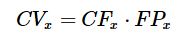### Error (Difference)

If error (difference) is the selected correction type then: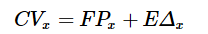### Error (% Actual)

If error (% actual) is the selected correction type then: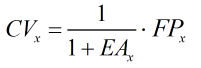If error (% reading) is the selected correction type then: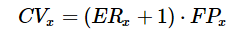If interpolate on correction factor is selected then the correction type is converted to a correction factor for interpolation.

### Correction Value

For a correction value this is done by: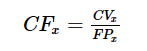### Error (Difference)

If error (difference) is the selected correction type then: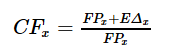### Error (% Actual)

If error (% actual) is the selected correction type then: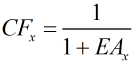If error (% reading) is the selected correction type then: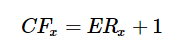Where FPx = Reference values CVx = Correction values corresponding to footprint CFx = Correction factors corresponding to footprint EΔ = Error (difference) values corresponding to footprint EAx = Error (% actual) values corresponding to footprint ER = Error (% reading) values corresponding to footprint

### Corrected Output

The corrected output is calculated using linear interpolation.

Back